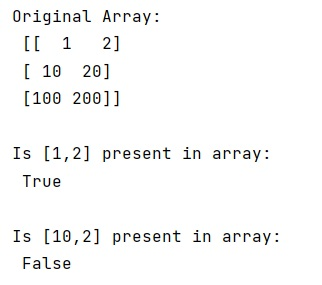# Check whether a Numpy array contains a specified row

Learn, how to check whether a Numpy array contains a specified row in Python?
Submitted by Pranit Sharma, on January 21, 2023

NumPy is an abbreviated form of Numerical Python. It is used for different types of scientific operations in python. Numpy is a vast library in python which is used for almost every kind of scientific or mathematical operation. It is itself an array which is a collection of various methods and functions for processing the arrays.

## Checking whether a Numpy array contains a specified row

Suppose that we are given a NumPy array and we need to check whether a Numpy array contains at least one instance of a given row. It must terminate upon finding the first matching row rather than iterating over the entire array even if a result has already been found.

For this purpose, we will use the tolist() method with our NumPy array and check whether the given row is present in the array or not.

Let us understand with the help of an example,

## Python code to check whether a Numpy array contains a specified row

```# Import numpy
import numpy as np

# Creating a numpy array
arr = np.array([[1,2],[10,20],[100,200]])

# Display original array
print("Original Array:\n",arr,"\n")

# Check that a rows is present in this array or not
res = [1,2] in arr.tolist()

# Display result
print("Is [1,2] present in array:\n",res,"\n")

# Check for another row
res = [10,2] in arr.tolist()

# Display result
print("Is [10,2] present in array:\n",res,"\n")
```

Output:What's New (MCQs)

Top Interview Coding Problems/Challenges!

IncludeHelp's Blogs

Languages: » C » C++ » C++ STL » Java » Data Structure » C#.Net » Android » Kotlin » SQL
Web Technologies: » PHP » Python » JavaScript » CSS » Ajax » Node.js » Web programming/HTML
Solved programs: » C » C++ » DS » Java » C#
Aptitude que. & ans.: » C » C++ » Java » DBMS
Interview que. & ans.: » C » Embedded C » Java » SEO » HR
CS Subjects: » CS Basics » O.S. » Networks » DBMS » Embedded Systems » Cloud Computing
» Machine learning » CS Organizations » Linux » DOS
More: » Articles » Puzzles » News/Updates